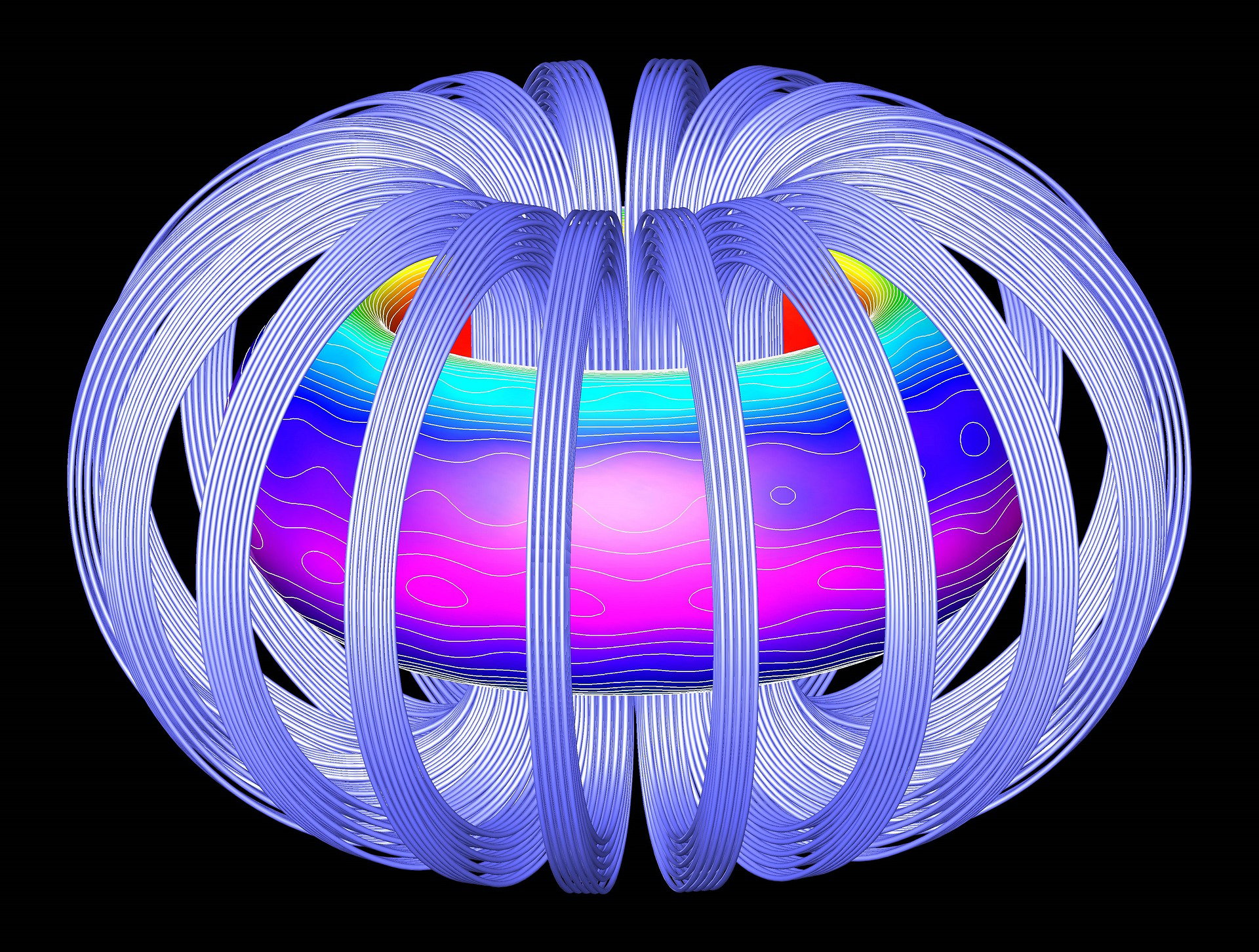STUDY AREAS

Measurement, Scalars, and Vectors

• Exponents, Graphing, Trigonometry Scalars and Vectors

Kinematics

• Distance and Displacement Speed and Velocity Acceleration
• The Kinematic Equations for Uniformly Accelerated Motion
• Graphs of Motion

Dynamics

• Newton’s First Law of Motion
• Newton’s Second Law of Motion
• Newton’s Third Law of Motion

Momentum and Impulse

• Momentum
• Impulse
• Conservation of Momentum in One Dimension

Work and Energy

• Work
• Energy
• Conservation of Energy Power

Circular Motion and Rotation

• Uniform Circular Motion
• Torque
• Angular Momentum

Vibrations

• The Dynamics of Harmonic Motion
• Energy Considerations in Harmonic Motion

Gravity

• Newton’s Law of Universal Gravitation
• Orbiting Satellites

Electric Fields, Forces, and Potentials

• Charge
• Coulomb’s Law
• Separation and Transfer of Charge in an Electroscope Electric Field

Circuits

• Current, Voltage, and Resistance
• Power and Joule’s Law of Heating
• Circuits with More Than One Resistor Capacitance and Resistance-Capacitance Circuits

Magnetic Fields and Forces

• Magnetism
• Electromagnetic Induction

General Wave Properties

• Basic Properties of Mechanical Waves
• Sound Waves and the Doppler Effect
• Reflection, Refraction, Diffraction, and Interference of Mechanical Waves
• Standing Waves

Reflection and Refraction of Light

• Electromagnetic Waves
• Reflection of Light
• Refraction of Light
• Total Internal Reflection

Polarization, Diffraction, and Interference of Light

• Polarization Diffraction and Interference

Ray Optics

• Mirrors
• Lenses

Heat, Temperature, and Thermodynamics

• Heat and Temperature Specific Heat and Heat Capacity Phase Changes
• The First and Second Laws of Thermodynamics

Gases and Kinetic Theory

• Ideal Gases The Kinetic Theory of Gases

Quantum Phenomena

• Photons and the Photoelectric Effect
• The Momentum of a Photon and the Heisenberg Uncertainty
• Principle Matter Waves and the de Broglie Wavelength of a Particle

Atomic Physics

• Rutherford Model of the Atom
• Bohr Model of the Atom

Nuclear and Particle Physics

• Nuclear Structure
• Nuclear Binding Energy
• Nuclear Reactions

Special Relativity

• The Two Postulates in the Theory of Special Relativity

TEXTS:

Electric potential energy (Ep) is the energy that is needed to move a charge against an electric field. More energy is needed to move a charge further in the electric field and to move it through a stronger electric field.

A charge will exert a force on any other charge in the field and potential energy arises from any collection of charges. It is directly proportional to the value of charges (q1, q2) and inversely proportional to the distance between them (r).

(k - 𝐸𝑝 = 𝑘 * Coloumb’s constant) 𝑞1*𝑞2𝑟

Electric potential (φ) is the electric potential energy per unit charge. To find the electric potential at a chosen spot, we are supposed to calculate how much the electrical potential energy of an imaginary positively charged particle (qo) would change if we moved it there. Positive charges move from regions of high potential to regions of low potential, while negative charges move from regions of low potential to regions of high potential.

φ = 𝐸𝑝𝑞𝑜 ⇒ φ = 𝑘 𝑞𝑟

Voltage (U) is the difference in potential energy per unit charge between two locations in an electric field. Therefore, it is a difference between the electric potentials of those two locations.

𝑈 = φ1 − φ2

The concept of electric potential energy, electric potential and voltage can be used in biology when explaining the transport of particles across the cell membrane, when it happens due to electric gradient. The membrane is held together by the attraction of opposite charges. It is possible to overcome this attraction by applying a large electric potential across the membrane. In some cells, applied electric potentials are used to open and close the cell membrane in order to allow nutrients and waste to enter and exit the cell. In nerve cells, the electric potential across the membrane can be easily changed, allowing the cells to carry messages encoded in their membrane potential.

Circular motion is a movement of an object along the circumference of a circle or rotation along a circular path. Some of the physical quantities that characterize circular motion are: angular displacement, angular velocity, angular, tangential and centripetal acceleration, period and frequency. The angular displacement ( ) is the ratio of distance travelled around the circle ( S) Δθ Δ to the circle’s radius(r).Δθ = Δ𝑆𝑟Angular velocity (ω) is the measure of how an angle changes over time. It is the rotational analog of linear velocity (v) which is proportional to angular velocity times radius. Average angular velocity is proportional to the angular displacement and inversely proportional to time (t).𝑣 = ω * 𝑟 ω = ΔθΔ𝑡Since circular motion is more complex than linear, the body that is moving circulatory has three different kinds of accelerations: angular, tangential and centripetal. Angular acceleration (α) is defined as the rate of change of angular velocity. Tangential acceleration (at) is proportional to a change in the magnitude of the velocity over time. Tangential acceleration is linked to an increase or decrease in the velocity, but not its direction. Centripetal acceleration (ac) is the acceleration pointed towards the center of a curved path and perpendicular to the object’s velocity. It causes an object to change its direction and not its speed along a circular pathway. The centripetal acceleration has a magnitude equal to the square of the body’s velocity along the curve divided by the distance (r) from the center of the circle to the moving body.α = ΔωΔ𝑡 𝑎𝑡 = Δ𝑣Δ𝑡 𝑎𝑐 = 𝑣2𝑟Period (T) is the time needed for one revolution. Period is inversely proportional to angular speed.𝑇 = 2πωFrequency (ν) is the number of revolutions per second for a rotating object. It is inversely proportional to the period.ν = 1𝑇

Isaac Newton was the first scientist to precisely define the gravitational force and show that it could explain both falling bodies and astronomical motions. There were other scientists before Newton that suspected the same, but Newton was the first to propose an exact mathematical formula and to use it to explain the mechanism of astronomical motions.

Newton’s universal law of gravitation states that every object in the universe attracts every other object with a force that is directed along a line joining them. The force (F) is directly proportional to the product of their masses (m1m2) and inversely proportional to the square of the distance between them (r). The gravitational force is always attractive.( is gravitational constant, 𝐹 = γ its value is ) 𝑚1𝑚2𝑟2 γ 6, 67 * 10−11 𝑁*𝑚2𝑘𝑔2

Therefore, objects fall because the Earth’s gravitational force is pulling them towards it. Heavenly bodies are also pulled towards each other with a gravitational force that is acting as a centripetal force. The inertia of the body would cause it to tend to follow a straight-line path, but the centripetal gravitational force pulls it toward the center of the orbit of another body.

There is a popular legend that Newton suddenly discovered the law of universal gravitation when an apple fell from a tree and hit him on the head. A more probable explanation is that he was walking through an orchard and wondered why all the apples fell in the same direction with the same acceleration.

Thermodynamics is a branch of physics that deals with the relationships between heat and other forms of energy. It describes the transfer of energy from one place to another and from one form to another. The fundamental principles of thermodynamics are expressed in four laws: Zeroth, First, Second and Third Law Of Thermodynamics.

The Zeroth Law states that if two bodies are in thermal equilibrium with a third body, then they are also in equilibrium with each other.

The First Law states that the amount of heat received by the system (Q) is equal to the sum of the work of the system (A) and the change in its internal energy (ΔU). Therefore, it is impossible to construct a machine that would work forever without consuming any form of energy.

𝑄 = 𝐴 + Δ𝑈

The Second Law states that heat energy cannot be transferred from a body at a lower temperature to a body at a higher temperature without the addition of energy. It’s because when there is a change in temperature, there must be a change in internal energy of the system, as well. In other words, there is no thermodynamic process in which the only result would be the transformation of heat into useful work.

𝑄 ≠ 𝐴

The Third Law states that the entropy at absolute zero is zero. Entropy (S) is a measure of the disorder in a system, and absolute zero is a temperature of 0 K. There can be no physical system with negative entropy, so entropy always has a positive value or is equal to zero.

𝑆 ≥ 0

In physics, a wave is a disturbance that travels through space and matter transferring energy from one place to another. Depending on whether they need a medium (matter to travel through), waves can be mechanical or electromagnetic. Mechanical waves are waves that require a medium. These waves travel when molecules in the medium collide with each other passing on energy. Electromagnetic waves are waves that don't need a medium or matter. They travel through electrical and magnetic fields that are generated by charged particles. Waves can also be divided based on the direction of the wave. Transverse waves are waves in which the medium moves at right angles to the direction of the wave. Transverse waves have two parts: crest (the highest point of the wave) and trough (the lowest point of the wave). Compressional (or longitudinal)waves are waves in which the medium moves back and forth in the same direction as the wave. Compressional waves have two parts, as well: compression (where the particles are close together) and rarefaction (where the particles are spread apart).Some important wave properties are: the amplitude, the wavelength, the frequency, the period and the wave speed. The amplitude of a wave is a measure of the displacement of the wave from its rest position. It shows us the intensity of the wave. The wavelength (λ) of a wave is the distance between one point on a wave and the exact same place on the next wave. The frequency (f) of a wave is the number of times per second that the wave cycles. Frequency is measured in Hertz or cycles per second. The higher the frequency, the closer the waves are together and the greater the energy carried by the waves will be. The period (Τ) of the wave is the time between wave crests. Period and frequency are reciprocals of each other. The wave speed (v) shows us how fast the disturbance of the wave is moving. It can be calculated by multiplying the frequency by the wavelength. The speed of mechanical waves depends on the medium that the wave is traveling through.       𝑇 = 1𝑓 𝑣

Magnetic field is the area around a magnet, magnetic object, or an electric charge in which magnetic force is exerted. Magnetic fields are created by moving electric charges. These fields can be created inside the atoms of magnetic objects or within current-carrying wires. The strength of a magnetic field (B) depends on the permeability of free space (μo), magnitude of the electric current (I) and the distance (r) between the source of the magnetic field and the point in which we are measuring its strength. The strength of a magnetic field is measured in Teslas.

(T).𝐵 = μ𝑜*𝐼2π𝑟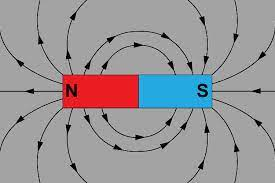Field lines of a magnetic field are directed from its north to its south pole. Field lines travel in “closed paths,” which means they will continue to travel the same path again and again. If we place a current-carrying wire in an external magnetic field, it will experience a magnetic force. Magnetic force is proportional to the magnitude of electric current (I), the length of a wire (L), and the strength of the magnetic field (B). It also depends on the angle between the length of wire and the magnetic field (α).𝐹 = 𝐼𝐿𝐵𝑠𝑖𝑛αIt is also possible to generate a current by moving a magnet through a coil of wire. This is called electromagnetic induction and this principle is the basis for a generator, which, this way, converts mechanical energy into electrical energy.

Energy (E) is defined as the capacity for doing work. Whenever the work is done there is a transfer of energy between bodies, environments, or body and environment. Energy can take a number of different forms. It can be chemical, electrical, gravitational, nuclear, kinetic, potential, etc. Energy can’t be created or destroyed, it only changes from one form to another, which states The Law Of Conservation Of Energy. Energy is measured in joules (J). One joule is equal to the work done by a force of one newton(N) acting over a distance of one meter (m).

[𝐸] = 𝐽 = 𝑁𝑚

When we are describing the movement or position of a body, we are usually dealing with mechanical energy. Mechanical energy can be either kinetic energy, potential energy, or the sum of these two. Kinetic energy is the energy of motion. Every moving object has kinetic energy. Kinetic energy is directly proportional to the square of its speed and the mass of the object.

𝐸𝑘 = 𝑚*𝑣22

Potential energy is the stored energy of position possessed by an object. When the object is in the gravitational field, we are dealing with gravitational potential energy. It is the energy stored in an object as the result of its vertical position or height. Gravitational potential energy is directly proportional to the mass and the height of an object.

𝐸𝑝 = 𝑚 * 𝑔 * ℎ(g - gravitational acceleration; g = 9.81 ) 𝑚𝑠2

Radioactive decay is the process that occurs when an unstable atomic nucleus releases energy in the form of emitted particles or electromagnetic waves. Atoms that produce radioactive decay are called radionuclides.There are many types of emitted particles or electromagnetic waves that elements can radiate. The three pivotal types are: alpha, beta, and gamma.

An alpha particle (α) is made up of two protons and two neutrons bound together. It has the same structure as a helium atom missing its two electrons, and therefore it has a positive charge. Alpha decay is the process that happens because the nucleus of an element has too many protons. A nucleus with too many protons causes repulsion between these charges, and to reduce it, the nucleus emits an α particle.

Beta particle (β) is an electron released from an atom. The process of beta decay takes place in radioactive nuclei with too many neutrons. To decrease the number of neutrons, one neutron converts into a proton, and thereby releases an electron as a beta particle. After alpha and beta decay the atom has changed into a diﬀerent element since the number of protons before and after the decay is diﬀerent (the number of protons defines each element).

Gamma rays (γ) are electromagnetic waves that are released by some decay reactions. Gamma radiation has no mass or charge. Gamma decay reactions eventuate if the energy of the element’s nucleus is too high. The resulting atomic number and atomic mass of an element during gamma decay remain unchanged.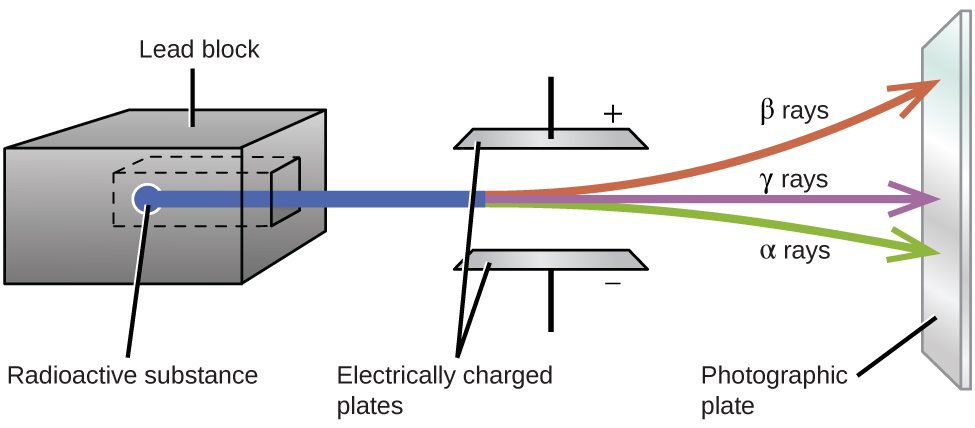Elasticity is the property of solid materials to return to their original shape and size after the force deforming them has been removed. Consequently, an elastic body is one that returns to its original shape after a deformation. A spring is an example of an elastic body. Elastic bodies exert a counter force in the direction that is opposite to the direction of the stretching. This force is called elastic force. The farther the material is stretched or compressed, the greater the elastic force becomes. As soon as the stretching or compressing force is released, elastic force causes the material to go back to its original shape. Hooke's Law is a principle of physics that states that the force needed to extend or compress a spring by some distance is proportional to that distance. The law is named after the British physicist Robert Hooke, who attempted to demonstrate the relationship between the forces applied to a spring and its elasticity.

F = k*Δx

(F represents the elastic force, Δx is the difference between the original length and the stretched length of the spring, and k is a spring constant that refers to the stiffness of the spring.)

A lens is a carefully molded piece of transparent material that refracts light rays in such a way  to form an image. The lens has its principal (horizontal) and vertical axis.

There are two types of lenses based on the way they refract light: converging and diverging lenses.

A converging lens is a lens that converges rays of light that are traveling parallel to its principal axis. They are thick across their middle and thin at their upper and lower edges. They are also called concave lenses.

A diverging lens is a lens that diverges rays of light that are traveling parallel to its principal axis. They are thin across their middle and thick at their upper and lower edges. They are also called concave lenses.

The point where light rays converge when passing through a converging lens is known as the focal point (F) of the converging lens. On the other hand, if the light rays diverge, then the diverging rays can be traced backwards until they intersect at a point. This intersection point is known as the focal point (F) of a diverging lens. The distance from the lens to the focal point is known as the focal length (f). A lens does have an imaginary point that we refer to as the 2F point. This is the point on the principal axis that is twice as far from the vertical axis as the focal point is.

If we want to find out what kind of image will be formed by the lens, we can draw two rays from the object whose image is being formed: one ray entering the lens parallel to the principal axis and bending through the focal point, and another ray that passes straight through the center of the lens without bending. The image is formed at the location of the intersection of these two rays.

Kinetics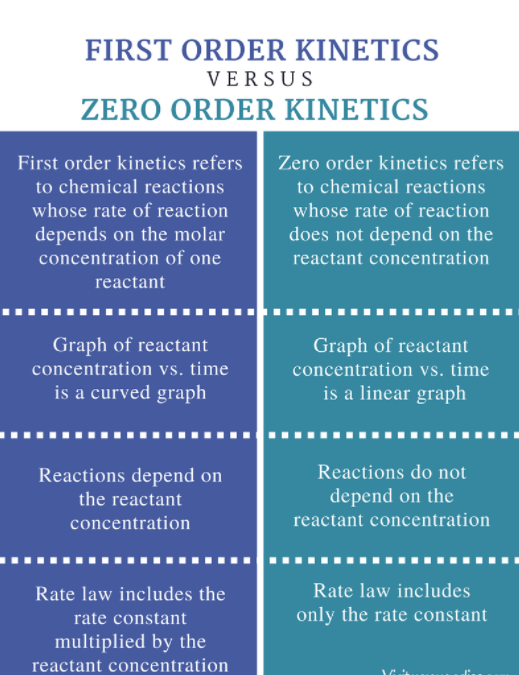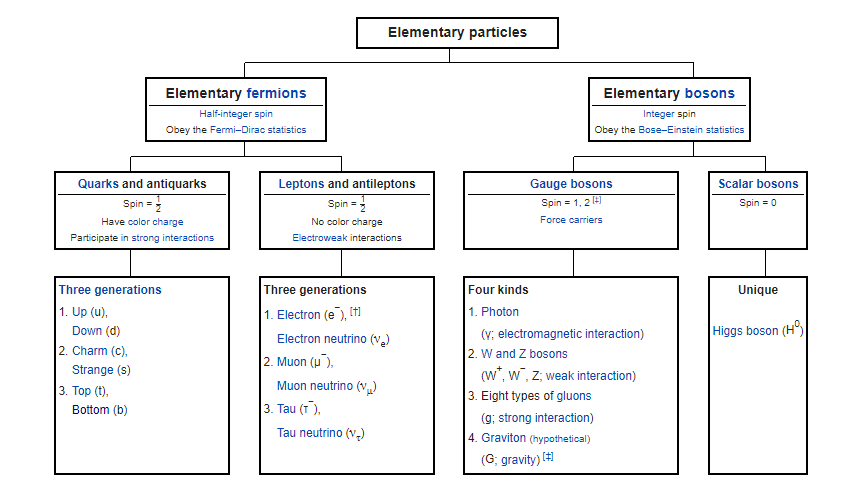QUESTIONS FOR DISSCUSION AND CONVERSATION:

Can a fire have a shadow?

Can gold be created from other elements?

Can light bend around corners?

Can radio antennas emit visible light?

Can sound waves generate heat?

Could electronic devices charge themselves without being plugged into an electricity source?

Do atoms ever actually touch each other?

Does a source of electricity ever run out of electrons?

Does an atom have a color?

Does an electron in an atom move at all?

Does time go faster at the top of a building compared to the bottom?

How can a clear object be transparent and visible at the same time?

How can a material at a certain temperature have all of its molecules at the same energy?

How can an electron leap between atomic levels without passing through all the space in between?

How can it be so hard to drag rubber across smooth glass if friction is caused by surface roughness?

How can we travel to the past?

How did Archimedes use mirrors to burn up invaders' ships?

How do airplanes fly upside down if it's the shape of the wings that make them fly?

How do free energy machines work?

How do levers create energy if the conservation of energy does not allow energy to be created?

How do projectors project the color black?

How do scientists cool objects to absolute zero?

How does a microwave oven heat up food even though it emits no thermal radiation?

How does a photon accelerate to light speed so quickly?

How does sound going slower in water make it hard to talk to someone underwater?

How high does a building have to be for a penny dropped from the top to kill a person on the ground?

How long can you use a cell phone before getting a brain tumor?

If I hammered and flattened a penny enough, could I cover the entire earth with it?

If I'm on an elevator that breaks loose and plummets down the shaft, can I avoid harm by jumping at the last second? Is it possible to create magnetic waves?

Is metal a good heat shield?

Is the reason that nothing can go faster than light because we have not tried hard enough?

Light has no mass so it also has no energy according to Einstein, but how can sunlight warm the earth without energy?

What are some other ways to save energy in a car besides turning off the air conditioner and rolling down the windows?

What did Schrodinger's Cat experiment prove?

What is the shape of an electron?

What is the speed of dark?

What is the speed of electricity?

What is the speed of gravity?

What is the strongest magnetic field possible?

What keeps a bicycle balanced?

What makes radioactive atoms get old so quickly and decay?

What makes the light waves in laser light parallel? What stops a piece of paper from being folded more than seven times?

What would happen if you drove your car close to the speed of light and turned on the headlights?

When I bump one end of a long metal bar, the other end instantaneously moves.

Can I use this to send messages faster than light?

When I step out of the shower, what makes the tile floor so much colder than the bathroom mat?

Are all metals magnetic?

Why are sound waves invisible? Why do mirrors flip left to right and not up to down?

Why does a rainbow contain a pure spread of spectral colors?

Why does a rainbow exist only in a narrow band?

Why does lightning push electricity through air, but common batteries do not?

Why does my room get dark when I turn the lights off even if my window is shut? Why does water make a shirt darker?

Why don't atoms collapse if they are mostly empty space?

Why don't electrons in the atom enter the nucleus?

Why don't I feel the miles of air above me that are crushing me down?

Why is a 12-volt household battery harmless, but the shock from a 12-volt car battery will kill you?

Why is physics scale invariant? Why was color invented by humans?

Why were electrons chosen to be negatively charged?

Wouldn't it make more sense to call electrons positively charged because when they move they make electricity?

Why will a delivery truck filled with birds sitting on its floor be heavier than a truck with the same birds flying around inside.